# KSEEB Solutions for Class 6 Maths Chapter 11 Algebra Ex 11.1

Students can Download Chapter 11 Algebra Ex 11.1 Questions and Answers, Notes Pdf, KSEEB Solutions for Class 6 Maths helps you to revise the complete Karnataka State Board Syllabus and score more marks in your examinations.

## Karnataka State Syllabus Class 6 Maths Chapter 11 Algebra Ex 11.1

Question 1.
Find the rule which gives the number of matchsticks required to make the following matchstick patterns. Use a variable to write the rule,
Solution:
a) A pattern of letter T as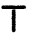From the figure, it can be observed that it will required two matchsticks to make a T, Therefore the pattern is 2n

b) A pattern of letter Z as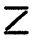From the figure, it can be observed that it will require three matchsticks two make a Z there fore, the pattern is 3n.

c) A pattern of letter U asFrom the figure, it can be observed that it will require there matchsticks to make a U. Therefore the pattern 3n

d) A pattern of letter V as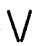From the figure it can be observed that it will required two matchsticks to make, a V, Therefore, the pattern is 2n

e) A pattern of letter E as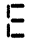From the figure, it can be observed that it will required five matchsticks to make an E. Therefore, the pattern in 5n.

f) A pattern of letter S as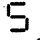From the figure, it can be observed that it will require five matchsticks to make a S therefore, the pattern is 5n

g) A pattern of letter A as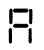From the figure, it can be observed that it will required six matchsticks to make an A, therefore, the pattern is 6n.Question 2.
We already know the rule for the pattern of letters L, C and F. Some of the letters from Q.1 (given above) give us the same rule as that given by L. Wgich are these? Why does this happen?
Solution:
It is known that L required only two matchsticks Therefore the pattern for L is 2n Among all the letters given above in question 1, only T and V are the two letters Which require two matchsticks Hence (a) and (b).Question 3.
Cadets are marching in a parade. Therefore are 5 cadets in a row. What is the rule which gives the number of cadets, given the number of rows? (Use n for the number of rows.)
Solution:
Let number of rows be n
Number of cadets in one row = 5
Total number of cadets = number of cadets in a row x numbers of rows= 5

Question 4.
If there are 50 managoes in a box, how will you write he total number of managoes in terms of the number of-boxes?(Use b for the number of boxes).
Solution:
Let the number of boxes b b
Number of manager in a box = 50
Total number of managoes = Numbers of mangoes in a box × numbers of boxes = 50b

Question 5.
The teacher distributes 5 pencils per students can you tell hoe many pencils are needed given the number of students? (use S for the number of students)
Solution:
Let the number of students be S pencils given to each student = 5
Total number of pencils = Number of pencils given to each students × number of students,
= 5sQuestion 6.
A bird flies 1 kilometer in one minute. Can you express the distance covered by the bird in terms of its flying time in minutes?(Use t for flying time in minutes).
Solution:
Let the flying time be t minutes
Distance covered in one minute = 1 km
Distance covered n t minutes = Distance covered in one minute × Flying time
= 1 × t = t km

Question 7.
Radha is drawing a dot Rangoli ( a beautiful pattern of lines joining dots with chalk powder, she has 9 dots in a row. How many dots will her Rangoli have for r rows? How many dots are there if there are 8 rows? if there are 10 rows?Solution:
Number of dots in 1 row = 9
Number of rows = r
Total number of dots in r rows = Number of rows × number of dots in a row
= 9r
Number of dots in 8 rows = 8 × 9 = 72
Number of dots in 10 rows = 10 × 9 = 90Question 8.
Leela is Radha’s younger sister. Leela is 4 years younger than Radha. can you write Leele’s age in terms of Radha’s age? Take Radha’s age to be x years.
Solution:
Let Radha’s age be x years
Leele’s age = Radha’s age – 4
= (x – 4) years.

Question 9.
Solution:
Number of laddus given away = 1
Number of laddus remaining = 5
1 + 5Question 10.
Orange are to be transferred from larger boxes into smaller boxes. When a large box is emptied, the oranges from it fill two smaller oxes and still 10 oranges remain outside, if the number of oranges in a small box are taken to be x, what is the number of oranges in the larger box?
Solution:
Number of oranges in one small box = x
Number of orange in two small boxes = 2x
Number of orange left = 10.
Number of orange in the large box =
Number of orange in two small boxes + Number of orange left = 2x + 10

Question 11.
a) Look at the following matchstick pattern of squares (Fig 11.6). The squares are
not separate. Two neighbouring squares have a common matchstick. observe the patterns and find the rule that gives the number of matchsticks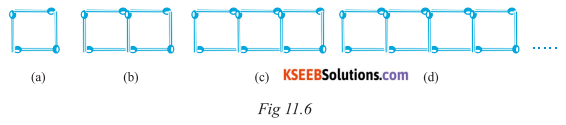in terms of the number of squares( Hint: if you remove the vertical stick at the end. you will get a pattern of Cs.)
b) Fig 11.7 gives a matchstick pattern of triangles. As in Exercise 11(a) above, find the general rule that gives the number of matchsticks in terms of the number of triangles.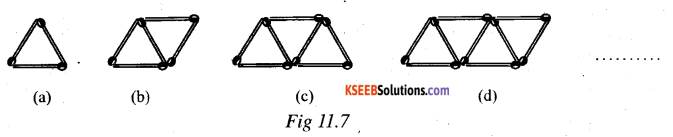Solution:
a) If can be observed that in the given matchsticks pattern, the number of matchsticks are 4, 7, 10 and 13. which is 1 more than thrice of the numbers of square in the pattern. Hence, the pattern is 3n +1 Where n is the number of squares.
b) It can be observed that in the given matchsticks pattern, the number of matchstick pattern the number are 3, 5, 7 and 9. which is 1 more than twice of the number of ∆ les in the pattern
Hence, the pattern is 2n + 1 Where n is the number of ∆les.